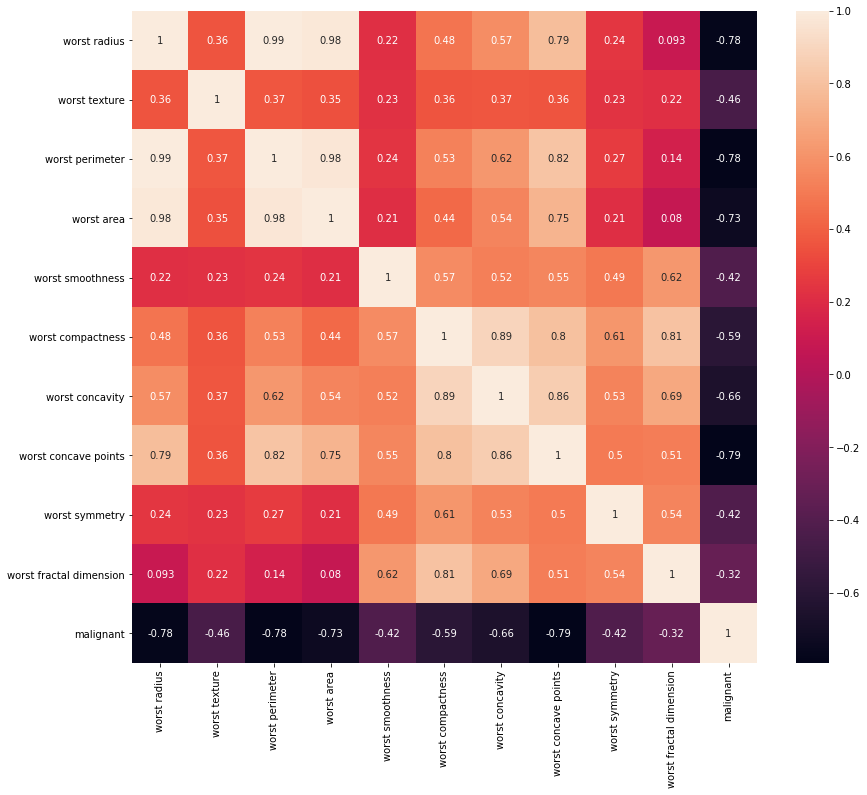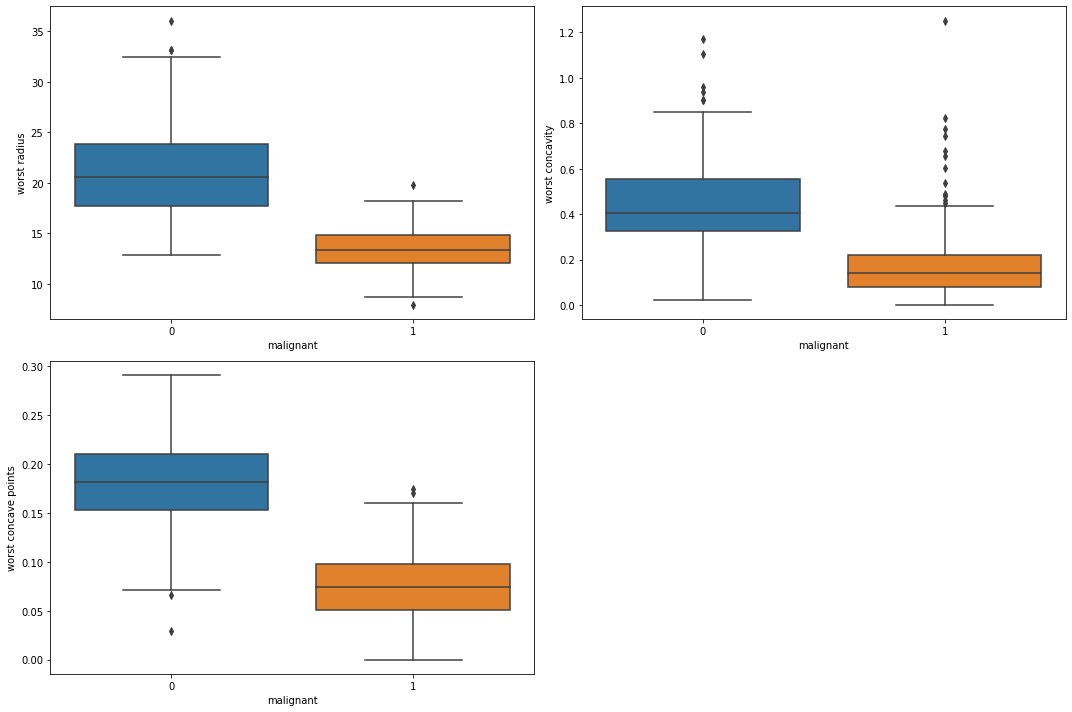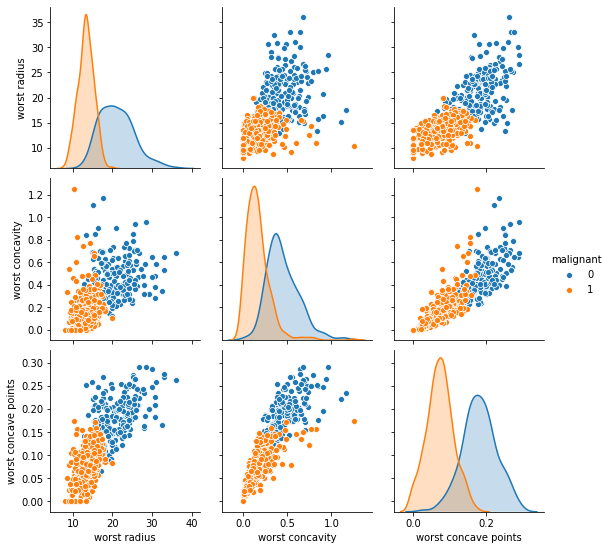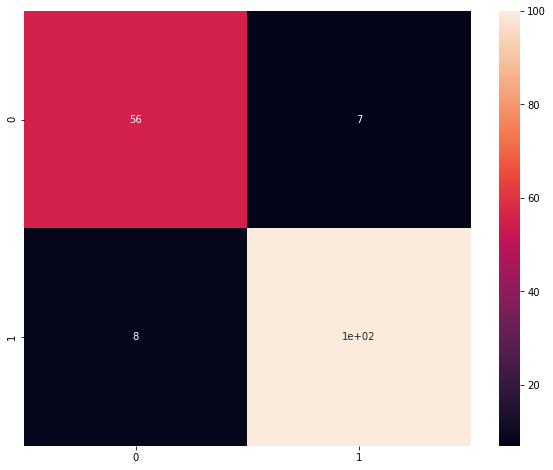# Hands-On: Supervised Learning - Classification¶

### AI and Predictive Analytics in Data-Center Environments - http://dcai.bsc.es¶

In this hands-on we continue with the topic of Supervised Learning, this time we will use a Naive Bayes classifier to decide in which one of two classes belongs each one of a set of observations. As usual the first step is to import the required packages:

In :
import numpy as np
import pandas as pd
import matplotlib.pyplot as plt
import seaborn as sns
import sklearn


For this exercise we will use the Breast Cancer dataset, also included in Sklearn's datasets package:

In :
from sklearn.datasets import load_breast_cancer


Let's take a look at the description of the dataset so we can get a better idea of what's in it:

In :
print(breast_cancer_ds.DESCR)

.. _breast_cancer_dataset:

Breast cancer wisconsin (diagnostic) dataset
--------------------------------------------

**Data Set Characteristics:**

:Number of Instances: 569

:Number of Attributes: 30 numeric, predictive attributes and the class

:Attribute Information:
- radius (mean of distances from center to points on the perimeter)
- texture (standard deviation of gray-scale values)
- perimeter
- area
- smoothness (local variation in radius lengths)
- compactness (perimeter^2 / area - 1.0)
- concavity (severity of concave portions of the contour)
- concave points (number of concave portions of the contour)
- symmetry
- fractal dimension ("coastline approximation" - 1)

The mean, standard error, and "worst" or largest (mean of the three
largest values) of these features were computed for each image,
resulting in 30 features.  For instance, field 3 is Mean Radius, field

- class:
- WDBC-Malignant
- WDBC-Benign

:Summary Statistics:

===================================== ====== ======
Min    Max
===================================== ====== ======
texture (mean):                       9.71   39.28
perimeter (mean):                     43.79  188.5
area (mean):                          143.5  2501.0
smoothness (mean):                    0.053  0.163
compactness (mean):                   0.019  0.345
concavity (mean):                     0.0    0.427
concave points (mean):                0.0    0.201
symmetry (mean):                      0.106  0.304
fractal dimension (mean):             0.05   0.097
texture (standard error):             0.36   4.885
perimeter (standard error):           0.757  21.98
area (standard error):                6.802  542.2
smoothness (standard error):          0.002  0.031
compactness (standard error):         0.002  0.135
concavity (standard error):           0.0    0.396
concave points (standard error):      0.0    0.053
symmetry (standard error):            0.008  0.079
fractal dimension (standard error):   0.001  0.03
texture (worst):                      12.02  49.54
perimeter (worst):                    50.41  251.2
area (worst):                         185.2  4254.0
smoothness (worst):                   0.071  0.223
compactness (worst):                  0.027  1.058
concavity (worst):                    0.0    1.252
concave points (worst):               0.0    0.291
symmetry (worst):                     0.156  0.664
fractal dimension (worst):            0.055  0.208
===================================== ====== ======

:Missing Attribute Values: None

:Class Distribution: 212 - Malignant, 357 - Benign

:Creator:  Dr. William H. Wolberg, W. Nick Street, Olvi L. Mangasarian

:Donor: Nick Street

:Date: November, 1995

This is a copy of UCI ML Breast Cancer Wisconsin (Diagnostic) datasets.
https://goo.gl/U2Uwz2

Features are computed from a digitized image of a fine needle
aspirate (FNA) of a breast mass.  They describe
characteristics of the cell nuclei present in the image.

Separating plane described above was obtained using
Multisurface Method-Tree (MSM-T) [K. P. Bennett, "Decision Tree
Construction Via Linear Programming." Proceedings of the 4th
Midwest Artificial Intelligence and Cognitive Science Society,
pp. 97-101, 1992], a classification method which uses linear
programming to construct a decision tree.  Relevant features
were selected using an exhaustive search in the space of 1-4
features and 1-3 separating planes.

The actual linear program used to obtain the separating plane
in the 3-dimensional space is that described in:
[K. P. Bennett and O. L. Mangasarian: "Robust Linear
Programming Discrimination of Two Linearly Inseparable Sets",
Optimization Methods and Software 1, 1992, 23-34].

This database is also available through the UW CS ftp server:

ftp ftp.cs.wisc.edu
cd math-prog/cpo-dataset/machine-learn/WDBC/

.. topic:: References

- W.N. Street, W.H. Wolberg and O.L. Mangasarian. Nuclear feature extraction
for breast tumor diagnosis. IS&T/SPIE 1993 International Symposium on
Electronic Imaging: Science and Technology, volume 1905, pages 861-870,
San Jose, CA, 1993.
- O.L. Mangasarian, W.N. Street and W.H. Wolberg. Breast cancer diagnosis and
prognosis via linear programming. Operations Research, 43(4), pages 570-577,
July-August 1995.
- W.H. Wolberg, W.N. Street, and O.L. Mangasarian. Machine learning techniques
to diagnose breast cancer from fine-needle aspirates. Cancer Letters 77 (1994)
163-171.


This dataset has 30 features, which is way more than in any of our previous examples! We will have then to perform some feature selection to avoid having an overly complicated model. If we take a look at the description we can see that there are only 10 distinct attributes, with 3 statistics (mean, worst case and standard error) calculated for each one. To simplify our problem we will check if we can get reasonable results by taking only the worst case. Let's then put the data into a pandas DataFrame and select the features we are interested in:

In :
breast_cancer_df_raw = pd.DataFrame(data=breast_cancer_ds.data, columns=breast_cancer_ds.feature_names)
worst_cols = filter(lambda x: x.split() == "worst", breast_cancer_ds.feature_names)
breast_cancer_df = pd.DataFrame(breast_cancer_df_raw[worst_cols])

Out:
worst radius worst texture worst perimeter worst area worst smoothness worst compactness worst concavity worst concave points worst symmetry worst fractal dimension
0 25.38 17.33 184.60 2019.0 0.1622 0.6656 0.7119 0.2654 0.4601 0.11890
1 24.99 23.41 158.80 1956.0 0.1238 0.1866 0.2416 0.1860 0.2750 0.08902
2 23.57 25.53 152.50 1709.0 0.1444 0.4245 0.4504 0.2430 0.3613 0.08758
3 14.91 26.50 98.87 567.7 0.2098 0.8663 0.6869 0.2575 0.6638 0.17300
4 22.54 16.67 152.20 1575.0 0.1374 0.2050 0.4000 0.1625 0.2364 0.07678

Now that we have selected our data, let's add the labels to the dataset to ease its manipulation:

In :
breast_cancer_df['malignant'] = breast_cancer_ds.target

Out:
worst radius worst texture worst perimeter worst area worst smoothness worst compactness worst concavity worst concave points worst symmetry worst fractal dimension malignant
0 25.38 17.33 184.60 2019.0 0.1622 0.6656 0.7119 0.2654 0.4601 0.11890 0
1 24.99 23.41 158.80 1956.0 0.1238 0.1866 0.2416 0.1860 0.2750 0.08902 0
2 23.57 25.53 152.50 1709.0 0.1444 0.4245 0.4504 0.2430 0.3613 0.08758 0
3 14.91 26.50 98.87 567.7 0.2098 0.8663 0.6869 0.2575 0.6638 0.17300 0
4 22.54 16.67 152.20 1575.0 0.1374 0.2050 0.4000 0.1625 0.2364 0.07678 0

We can now take a look to the correlation matrix to identify the most relevant feaures:

In :
plt.figure(figsize=(14, 12))
sns.heatmap(breast_cancer_df.corr(), annot=True);We can see that the majority of the features we selected have a quite high correlation value. For this example and for visualization purposes we will only select the one with the highest correlation value (either negative or positive), let's say over 0.65 in absolute value:

In :
most_relevant_features = breast_cancer_df.corr()[abs(breast_cancer_df.corr()["malignant"]) > 0.65].index.values
most_relevant_features

Out:
array(['worst radius', 'worst perimeter', 'worst area', 'worst concavity',
'worst concave points', 'malignant'], dtype=object)

The first three features selected are the radius, perimeter and area, which are quite correlated between them (as we can see in the correlation matrix). Because using the three of them will not add much more information than using just one, we will select only radius to keep the number of features to a minimum:

In :
most_relevant_features = ["worst radius", "worst concavity", "worst concave points", "malignant"]
breast_cancer_short_df = breast_cancer_df[most_relevant_features]

Out:
worst radius worst concavity worst concave points malignant
0 25.38 0.7119 0.2654 0
1 24.99 0.2416 0.1860 0
2 23.57 0.4504 0.2430 0
3 14.91 0.6869 0.2575 0
4 22.54 0.4000 0.1625 0

Now that we have identified the most relevant features we can check if there's a significant value separation between classes for each one of them using a boxplot:

In :
import math
plt.figure(figsize=(15,10))
for i, feature in enumerate(breast_cancer_short_df.columns[:-1]):
rows = math.ceil(len(breast_cancer_short_df.columns[:-1])/2)

plt.subplot(rows, 2, i+1)

sns.boxplot(x="malignant", y=feature, data=breast_cancer_short_df)

plt.tight_layout()
plt.show()There is indeed a significant value separation between classes, so we can expect to get a reasonably good classification with a relatively simple classifier. Let's now plot the scatter matrix of the selected features:

In :
sns.pairplot(breast_cancer_short_df,vars=breast_cancer_short_df.columns[:-1], hue="malignant");Although there is some overlap, we can see here some clear separation.

Before starting with our model definition let's split the data two for training and validation purposes:

In :
from sklearn.model_selection import train_test_split
train_df, test_df = train_test_split(breast_cancer_short_df, test_size=0.3, random_state=0)


And get the names of the features and label column for convenience:

In :
feats = breast_cancer_short_df.columns[:-1]
label = breast_cancer_short_df.columns[-1]


In this occasion we will be using a Gaussian Naive Bayes classifier, which is provided by Sklearn. As with all Sklearn models, we can train it with the fit function provinding the training data and labels:

In :
from sklearn.naive_bayes import GaussianNB
gnb = GaussianNB()
gnb.fit(X=train_df[feats], y=train_df[label]);


And get the accuracy on the training data:

In :
train_score = gnb.score( train_df[feats], train_df[label])
print("Training accuracy: {}".format(train_score))
print("Test Accuracy: {}".format(gnb.score( test_df[feats], test_df[label] )))

Training accuracy: 0.9472361809045227
Test Accuracy: 0.9122807017543859


Once the model is trained, we can use it to make predicions using the predict method:

In :
y_pred = gnb.predict(test_df[feats]).T


And plot the confusion matrix:

In :
from sklearn.metrics import confusion_matrix
plt.figure(figsize = (10,8))
sns.heatmap(confusion_matrix(test_df[label], y_pred), annot=True);